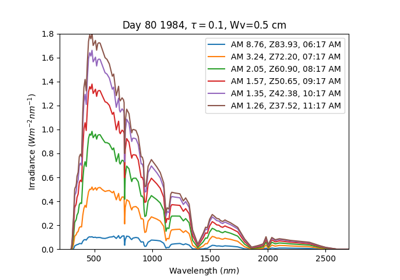# pvlib.solarposition.get_solarposition#

pvlib.solarposition.get_solarposition(time, latitude, longitude, altitude=None, pressure=None, method='nrel_numpy', temperature=12, **kwargs)[source]#

A convenience wrapper for the solar position calculators.

Parameters
• time (pandas.DatetimeIndex) – Must be localized or UTC will be assumed.

• latitude (float) – Latitude in decimal degrees. Positive north of equator, negative to south.

• longitude (float) – Longitude in decimal degrees. Positive east of prime meridian, negative to west.

• altitude (None or float, default None) – If None, computed from pressure. Assumed to be 0 m if pressure is also None.

• pressure (None or float, default None) – If None, computed from altitude. Assumed to be 101325 Pa if altitude is also None.

• method (string, default 'nrel_numpy') –

‘nrel_numpy’ uses an implementation of the NREL SPA algorithm described in  (default, recommended): `spa_python()`

’nrel_numba’ uses an implementation of the NREL SPA algorithm described in , but also compiles the code first: `spa_python()`

’pyephem’ uses the PyEphem package: `pyephem()`

’ephemeris’ uses the pvlib ephemeris code: `ephemeris()`

’nrel_c’ uses the NREL SPA C code : `spa_c()`

• temperature (float, default 12) – Degrees C.

• kwargs – Other keywords are passed to the solar position function specified by the `method` argument.

References

1

I. Reda and A. Andreas, Solar position algorithm for solar radiation applications. Solar Energy, vol. 76, no. 5, pp. 577-589, 2004.

2

I. Reda and A. Andreas, Corrigendum to Solar position algorithm for solar radiation applications. Solar Energy, vol. 81, no. 6, p. 838, 2007.

3

NREL SPA code: http://rredc.nrel.gov/solar/codesandalgorithms/spa/

## Examples using `pvlib.solarposition.get_solarposition`#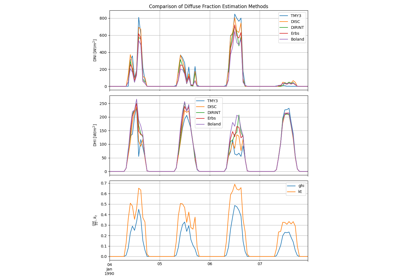Diffuse Fraction Estimation

Diffuse Fraction Estimation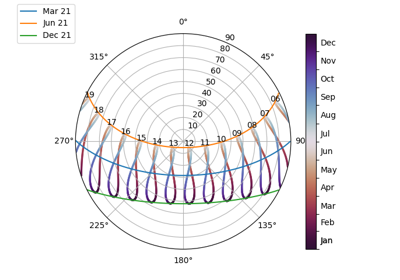Sun path diagram

Sun path diagram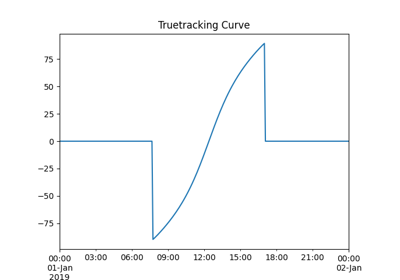Single-axis tracking

Single-axis tracking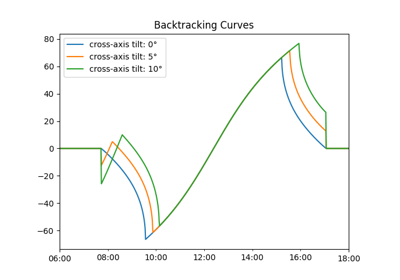Backtracking on sloped terrain

Backtracking on sloped terrain